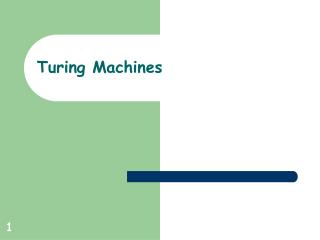Download PresentationTuring Machines

# Turing Machines

Download Presentation## Turing Machines

- - - - - - - - - - - - - - - - - - - - - - - - - - - E N D - - - - - - - - - - - - - - - - - - - - - - - - - - -
##### Presentation Transcript

1. Turing Machines

2. Formal Defn of a Turing Machine A TM is a 7-Tuple M = (Q, , , , q0, B, F) where • Q – Set of states •  – Input alphabet (B  ) •  – Tape alphabet (  and B  ) •  – Transition Function (partial function) •  : Q x   Q x  x {L, R} • q0 – Start state (q0  Q ) • B   Blank symbol • F  Q set of final states

3. Turing Machine Configurations • String uqv such that • u,v  * • uv is information on the tape • q is the current state • tape head is pointing to first symbol in v

4. Standard Turing Machine • No transitions out of accepting states • Halts when no transitions defined • Tape unbounded in both directions • Deterministic: ≤ 1 transition per configuration • Input: content of tape at start • Output: content of tape when halted

5. Taking Steps in a Turing Machine • For a,b,c   and u,v  * and qi,qj  Q • uaqibv ├ uacqjv if (qi,b) = (qj,c,R) • uaqibv ├ uqjacv if (qi,b) = (qj,c,L)

6. Starting, Accepting and Rejecting • For M = (Q, , , , q0, , F) • Start configuration of input w * • q0w • Accepting configuration • Halt in configuration containing q F • Rejecting configuration • Halt in configuration containing q  F

7. Computation • Sequence from a start configuration to a halting configuration

8. Infinite Loop • Never reach a halting configuration for some start configuration C

9. Language of a Turing Machine • M accepts a string w iff there exists sequence of configuration C1, C2,...,Ck • C1 is the start configuration of M on input w • each Ci yields Ci+1 • Ck is an accepting configuration • L(M) = { w  * | M accepts w } • A language L is recursively-enumerable if L = L(M) for some Turing Machine M

10. Accepting, Rejecting and Looping • On input w TM M may • accept by halting in accept state • reject by halting in reject state • fail to accept by looping • Deciders – TMs that never loop

11. Turing-decidable Languages • L is recursive (or decidable) • if some TM decides it • if there exists TM M such that L = L(M) and M halts on all input.

12. Sets of Languages Recursively-enumerable Recursive Context-Free Regular